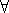## CGAL::Random_points_in_cube_3<Point_3, Creator>

### Definition

The class Random_points_in_cube_3<Point_3, Creator> is an input iterator creating points uniformly distributed in a half-open cube. The default Creator is Creator_uniform_3<Kernel_traits<P>::Kernel::RT,P>.

### Is Model for the Concepts

InputIterator
PointGenerator

#include <CGAL/point_generators_3.h>

### Types

 typedef std::input_iterator_tag iterator_category; typedef Point_3 value_type; typedef std::ptrdiff_t difference_type; typedef const Point_3* pointer; typedef Point_3 reference;

### Operations

 Random_points_in_cube_3 g ( double a, Random& rnd = default_random); g is an input iterator creating points of type Point_3 uniformly distributed in the half-open cube with side length 2 a, centered at the origin, i.e.p = *g: -ap.x(),p.y(),p.z() < a . Three random numbers are needed from rnd for each point.